## Friday, December 31, 2010

### ## On greenhouses and the Greenhouse Effect

From time to time, some people get excited about the fact that the greenhouse effect seems wrongly named. An extreme case is the paper of Gerlich and Tscheuschner, where they devote a whole section 2 (19 pages) to the question. It continues to arise; Judith Curry touched on it in a recent thread, where discussion turned to the old Wood's experiment

The standard response correctly says that none of this matters. The atmospheric  GHE is what it is, and is no less true if it turns out that the allusion to greenhouses is inaccurate. Wikipedia takes this approach, with a refreshing brevity and directness.

However, there is a little more to the story (which still says nothing about the truth of the GHE). Greenhouses do work mainly by blocking heat loss through convection. But IR flux blocking is not totally insignificant.

#### Wood's experiment

The description of this in the 1909 Philosophical Magazine is indeed brief. Some people give the impression that he built a greenhouse out of rock salt, but the geometry seems to be simpler and on a much smaller scale. Still, he concluded:
It seems to me very doubtful if the atmosphere is warmed to any great extent by absorbing the radiation from the ground, even under the most favourable conditions.

This final statement is little emphasised, but seems to be amply justified by the sketchiness of the note:
I do not pretend to have gone very deeply into the matter, and publish this note merely to draw attention to the fact that trapped radiation appears to play but a very small part in the actual cases with which we are familiar.

Indeed he didn't.

#### Modern Greenhouses

Ironically, the situation that forced Wood to use rock salt as an IR-permeable material is now reversed. In his day, greenhouses were always made of IR-blocking glass. Now they are often made of IR-permeable plastic. And, in support of Wood, they do work. They block convective heat transport, and also provide an insulating effect to limit conduction through the boundary.

However, a moderate amount of heat is still transferred by IR. Ironically, a reason why it isn't larger on balance is because of the countervailing downwelling IR, also a subject of occasional skepticism. Downwelling IR, from the atmosphere and especially clouds, is not that much less than upwelling. Glass blocks both upwelling and downwelling; the nett flux blocking is small, but if you are focussing on the fate of heat emanating from the surface, the IR fraction that is blocked us a larger fraction.

#### A real greenhouse issue

A covering that blocks IR actually makes a real improvement to greenhouse efficiency. It is more expensive, and people are willing to pay for it. Here are some industry observations:
NSW Ag Dept:
For example, films may be used to exclude ultra violet (UV) light for chemical free pest control or reflect long wave infra red (IR) radiation to improve heat retention at night. ... Long wave radiation (2500-40000 nm) absorbers reduce the loss of heat radiated from materials and objects (including plants) inside the greenhouse.

You can also buy a plastic film with an infrared inhibitor; it cuts heat loss inside the greenhouse by up to 20% on a cloudless night.

Actually, it would also cut loss on a clear day.

And here is a pamphlet which describes the practicality in some detail (for heated greenhouses, but the principle is the same).
The IR / anti-condensation treated films cost about \$0.015 per square foot more than untreated films but reduce energy use by 15 to 20%.

#### Scale Issues

The main thing wrong with the Wood's style reasoning is scale. IR blocking is a minor effect on the scale of a greenhouse because convection is relatively much more effective. The temperature gradients are huge compared to what is maintained in the atmosphere. Wood compounded this by experimenting on a much smaller scale again.

In the atmosphere, IR transport is more important than convection, so the blocking effect of GHG's matters much more.

.

## Friday, December 24, 2010

### ## Merry Christmas and happy holidays to all

It isn't freezing everywhere## Thursday, December 16, 2010

### ## Acidification

I should start with an apology for light posting lately. We have a family gathering with folks from overseas, but that wasn't the obstacle. We've been hassling to get some building work finished before the occasion (now done).

This post won't say much about the actual issue of ocean acidification. Rather it's a response to something that seems to always happen when the topic arises. Someone inevitably contends that, no, the ocean can't be acidifying because it's alkaline. pH>7 and all that.

There was a long discussion here, for example. What prompted me to post was seeing it happen recently on Judith Curry's blog. It's a sterile topic - a bit like arguing about whether the greenhouse effect is well named. But there's a bit of science in it. The argument can be answered on four levels.

#### The practical argument

The meaning is well-known. CO2 in solution will tend to dissolve calcium carbonate, thus disrupting life-forms. It may have other biological effects.

As with the greenhouse effect, human discourse doesn't require literal exactness. We can speak of currency inflation without arguing about whether dollar bills are getting bigger. etc.

#### The language argument

The objection is that you can't acidify something if it doesn't become "acid". But that just isn't normal usage. If you beautify something, it doesn't have to become beautiful. We can be enriched without becoming rich.

#### The theoretical chemistry argument

OK, getting more substantive. The notion that acidic is identified with pH<7 invokes an old notion of acidity. Since about 1923, the more general process going on in acid-base chemistry has been recognised as sharing an electron pair, rather than anything specific with protons. When sulphur trioxide reacts with calcium oxide to produce calcium sulphate, it is easy to recognise this as an acid-base reaction, with SO3 as the acid. No hydrogen is involved.

This is pretty much the case in the ocean. The overall reaction is something like:

CO2 + CaCO3 + H2O → Ca++ + 2HCO3-

Water is a reagent, and there may be a role for protons. But it isn't clear why pH 7 should matter in any way.

#### The aqueous chemistry argument - buffering

pH 7 is the neutral point of a particular acid-base equilibrium - in pure water. It also applies when a strong acid neutralises a strong base. But the ocean is not pure water, and does not have strong acids or bases.

A solution with substantial concentration of a weak acid and its corresponding base is described as buffered. That is because the pH is stabilised near the neutral point (pKa) of that equilibrium. If you add a strong acid, the protons will react with the corresponding base to produce more of the weak acid. Similar if you add a strong base. The pH changes little, even though real acid-base reaction occurs.

That's why pH 7 is irrelevant here. The ocean is dominated by a 3-way buffering involving CO2, bicarbonate and carbonate ions. There is a further solubility equilibrium between Ca++, CO3-- and solid calcium carbonate (eg aragonite). Adding CO2 pushes everything in the acid direction, which reduces CO3-- concentration and tends to dissolve CaCO3. It's a bit more complicated because the solubility equilibrium is not exactly attained - it is fairly easy for CaCO3 to be supersaturated in solution. But that is the direction.

## Wednesday, November 24, 2010

### ## New text and file repository

Over on the right, under Resources, there is a link to a "Document Store". That's where I keep various bits of code and other texts. The TempLS material is there, for example. I had been keeping it on a location on drop.IO.

Steve Mosher warned me that drop.IO has been taken over by Facebook, and will be closing. I looked around, and discovered that there is a much better alternative. Google Sites allows users, free, to set up a site with 100 Mb of space, to which you can upload files, images, etc. It also offers a range of templates replete with gadgets.

So I have set one up. It's a bit of a work in progress. The templates are impressive, but none quite matched. I chose a project template, which has lots of facilities, but some not really appropriate management talk. I've tried to keep that to a minimum.

So if you go to the new Document store you'll see a home page, with some intro stuff (not much yet), and a pointer to a page of "Project Documents". This is the new repository. The Google facility allows me much more freedom in writing descriptive text (so I'll have to do it :().

I'll also use it to host images - I've set up a "Picture Gallery". There's no real reason to look at that, as it's just for images to link in to posts, but you can if you want. I had been using TinyPic, but I don't need to do that any more.

I'll also transfer to it ongoing items like the category index of posts, and the temperature tracking page. I'll also make an updated TempLS page and keep it there.

The links within past posts still point to drop.IO, and I probably won't fix many of them. But you can always use the link under resources.

One merit of the new scheme is that I can link to individual files.

## Tuesday, November 23, 2010

### ## Woody Guthrie Award - thanks, Science of Doom

A note to thank Science of Doom. I am very honoured that he has chosen this blog  as his successor,  with the privilege of passing on the Woody Guthrie Award. I was especially pleased because SoD's style of dispassionate informative blogging is what I have aspired to here. So thanks, Science of Doom. And thanks to readers, who keep this blog going.

I did a bit of googling to find the history of the award, which I have been aware of since it first came to Skeptical Science. I've been able to track it back a few generations, as far back as Ã†rchie's Archive, who passed it on to Honji's Harangues. From there it went to honestpoet at
Enough is Enough, and she nominated Greenfyre's.

This was an interesting change, from a succession of rather philosophical blogs to one with a science/climate focus.

Greenfyre's passed it to Dan's Wild Wild Science Journal, who then passed it to
Skeptical Science. They awarded it to Science of Doom and, well, here we are.

Unfortunately, I can't find a reference to the event on Ã†rchie's Archive, so I can't go back further. But the search took me to a lot of interesting blogs, of which Archie's is one. It's discursive, but with a focus on Perth landscapes and history. I like Perth a lot - I've lived there - and I think Archie gets it well. Currently active and recommended.

The award used to come with a little graphic;which may have been more relevant in its earlier life cycle.

Anyway, it's now my job to pass it on. No need to rush there but thoughts would be welcome. Given its history, it seems ideas need not be restricted to the climate science community. I would have given a lot of thought to this excellent blog, but sadly, the owner retired a few months ago. I miss it.

## Saturday, November 13, 2010

### ## Condensation - the Eulerian View

In the previous post I analysed the effect of expansion in a cylinder on condensation. As the pressure reduces, of course, the volume increases. The key question was whether it increases more or less than if the air had been unsaturated.

This is supposed to help with what might happen in atmospheric uplift, as in a hurricane. The pressure reduces and the gas expands in the same way. If you focus on the moving air, in fluid mechanics that is the Lagrangian view.

It's better for intuition - the boundaries of the volume travel with the fluid, and by assumption in the atmosphere, heat and other species like water vapor travel with it too, and remain in the boundaries.. That is because diffusion over the large distances is relatively small, although thermal radiation could be an issue.

But there are problems when you want to think about the processes in relation to other things that are not moving with that fluid. Fixed boundaries are one issue - not so common in the atmosphere. But in the ongoing argument about Anastassia Makarieva's contraction theories (TaV1, JC and TaV2, Lucia1, Lucia2),  AM prefers the Eulerian frame, a fixed volume in space.

The good thing there is that you can assume steady conditions. At every point the velocity, temperature etc will remain steady, even though as you follow it in the flow, it changes. The bad thing is that the boundaries are no longer material boundaries, and you have to account for whatever is crossing it. Not so intuitive.

Scientists tend to talk Lagrangian to students, but Eulerian to their computers.

Here I'll take the Eulerian view of expansion and condensation.

#### A 1D (vertical) problem

In accounting for boundaries, it helps to have only two (upper and lower). I'll look at a 1 m thick slice, assuming zero horizontal gradients. The notation is generally as in the previous post. We'll say z goes from 0 to 1, and I'll suffix accordingly (but if suffix is missing, assume zero). So T,V,P, Ï are assumed known at z=0. The incoming air is saturated.

I'll also assume, as is conventional, hydrostatic pressure. So P1 =P0 -Ï g

Then each incoming packet of air changes according to the cylinder analysis, with this pressure increment:

T1 =T0 -Ï g dT/dP
where dT/dP = (T/P) DT/DP = (T/P) (1+c+c/b)/(3.5+b+b*c), b=L/(R*T), c = b*nw/n
In the previous post, n, nw were moles in a volume V. Here I'll take V=1, so they are molar densities.

nw1 =nw0 -Ï g dnw/dP
where dnw/dP = (nw/P) Dnw/DP = (nw/P) (1+c+c/b)/(3.5+b+b*c)

Density is calculated from dV. The mass change in the unit volume element Mw*dnw,  ( Mw = molar mass of water) so the density change is
d Ï /dP = Mw*dnw /dP + Ï/P DV/DP
= (Mw*nw /P *(b-3.5)+ Ï/P (2.5+b*c+2.5*c/b-c))/(3.5+c+b*c)

#### Velocity and precipitation reconciliation

The molar mass flux of dry air through z0 is    v0*Nd
The number of moles is conserved; in the notation of the previous post, Nd varies inversely as V, ie DNd/DP=-DV/DP.
So with v=1 m/s,
dv/dP=(v/P)Dv/DP = (v/P)DV/DP=(v/P)a4/a3
Velocity v increases with z.

The precipitation rate r moles/m3/s is
r=v dnw/dz= vÏ g (nw/P) (1+c+c/b)/(3.5+b+b*c)

#### Results

Again the meaningful test is to compare saturated air with unsaturated air under the same conditions. With z in km:
 dT/dz dnw/dz dÏ/dz dv/dz Dry -9.75 0 -0.0937 0.0818 Sat -4.151 -0.147 -0.1137 0.0969
The results are much as expected. dT/dz is the lapse rate. dnw/dz is numerically (withv=1) the precipitation rate in moles/km/m2/sec . 0.147 is equivalent to 9.5 mm/hr from a 1 km column - but remember the 1m/s velocity is arbitrary. The density reduces with height, but more rapidly for the saturated flow. And the velocity also increases more rapidly.

#### Interpretation

It is a steady flow. But also expanding. The upward velocity would have increased just because of the hydrostatic density gradient, but it increases more because of the condensation. This is due to the release of latent heat, which dominates the contraction due to mass loss.

The same expansion is seen in the density gradient (reducing faster). and the warming is seen in the well-known reduction of lapse rate with condensation.

#### Further note

I mentioned in the previous post that the formula for DT/DP could be found, with slight modification, in the Phys Met notes of Dr Caballero. I realised then that it is just the formula for the saturated adiabatic lapse rate, which has a much longer history.

As explained in that earlier post, my form isn't quite the same. There is a discrepancy due to the mole concentration I made in the Ideal Gas Law. I've kept with it, because it does seem to be the irght thing to do.

#### R Code

``` T=298.15
V=v=1
P=98131
R=8.314
N=P*V/(R*T)
Nw=0.03128*N
Cv=20.85
Mw=0.018
Ma=0.02896
L=2304900*Mw
g=9.8
rho = N*Ma

b=L/R/T
c=b*Nw/N
a1=1+c+c/b
a2=b-3.5
a3=b*a1-a2
a4= -(2.5*a1+c*a2)

dp=c(0,0,0,0)
dp=dTdP=(T/P)*(a1/a3)
dp=dNwdP=(Nw/P)*(a2/a3)
dp=drhodP  = (Mw*(Nw/P)*a2 - (rho/P)*a4)/a3
dp=(v/P)*a4/a3
dz=-dp*rho*g*1000
# print(dp); print(c(a1,a2,a3,a4))
print(dz)```
` `

## Saturday, November 6, 2010

### ## Condensation and expansion

There has been much blog discussion of whether condensation of water in the atmosphere is accompanied by a volume expansion or contraction. Standard theory says expansion, but Anastassia Makarieva has been promoting a theory that winds are driven by contraction caused by condensation. There is discussion here and here. The paper (M10) is available and open for online discussion here.

M10 has a whole section devoted to demonstrating that a fixed adiabatic volume cannot undergo condensation. As I'll show, this is an unsurprising result. But the section arises from reviews of an earlier paper suggesting hurricanes were driven by condensation-induced contraction. Reviewers objected that latent heat release would outweigh the tendency to contract to to loss of water vapor volume. So there was no contraction to provide energy.

On the Air Vent threads, various experiments to study condensation/contraction were proposed involving air in bags and bottles, etc. I have been trying to explain how they lack the adiabatic expansion that causes cloud condensation. Bodies of air rise, and as they do so they expand, doing work and cooling. If they are saturated with water vapor, some of this condenses. Because the cooling is adiabatic, the latent heat is retained and slows down the cooling and condensation process. The result is that the air parcel becomes warmer than its surroundings (though cooler than it was originally) and so expands relative to nearby air.

Below the jump, I'll describe a stationary volume-change process with condensation, and calculate the expansion effect.

Consider a volume of saturated air held adiabatically in a chamber with a piston which can force an expansion. For definiteness, let's say that  V=25 m3 at T=300°K, containing n=1000 moles of air+water at P=100 kPa. Latent heat (of vap) L=45 kJ/mol. There are nw =36 moles of water vapor present.

As the piston moves, there are three governing equations:
Conservation of energy
1)      n cv dT + L dnw + P dV = 0

Ideal Gas Law:
2)     P dV + V dP = n R dT + dnw RT

Clausius-Clapeyron Equation
3)     RT2 dnw = L nw dT (see update)

[Update - a small error here. I've identified  dnw/nw with dpw/pw  But in fact
pw =p(nw/n) .and p is not constant. So it should be
3)    RT2 (dnw/nw + dp/p)= L dT
It makes a small difference - full update to come (7.47 am 9 Nov)
It's here (140 pm) - I've marked new in brown, and obsolete in grey]

I prefer to put these in non-dimensional form, using a notation Dy =1/y dy (y=T,P etc). That means that Dy is a proportional change in y. So:

Divide (1) by PV or nRT
4)     cv/R DT + (L*nw)/(R*T*n) Dnw + DV = 0

Divide (2) by PV or nRT
5)      - DT - nw/n Dnw + DP + DV = 0

Divide (3) by R*T2*nw
6)      - L/(R*T) DT + Dnw + DP = 0

#### Solving

There are three equations in the four variables (DT, Dnw, DP, DV). However, one of these, DP or DV, is specified (the amount the piston is moved).

If you set DV=0, then there is just one solution DT= Dnw=DP=0. In other words, no condensation - nothing can happen. That's what Sec 2 of M10 was about.

Fixing DP is most natural for atmospheric comparison, since P is a measure of altitude. Dividing the equations by DP gives the derivatives ( DT/DP, Dnw/DP, DV/DP). Remember these are proportional derivatives. If you change P by p%, then you change V by (DV/DP)*p %.

I did a first solution with nw=0. This is in effect the dry air case. The R code is below.

With the diatomic cv/R = 5/2, it gave, as expected,
DT/DP=2/7=0.2857,    DV/DP=5/7= -0.714
That means that if P decreases by 1%, then the volume V increases by 0.71% and T decreases by 0.28%

Then I did the solution for the above case at 300 °K, 100 kPa. The answers were:
DT/DP=0.063,    Dnw/DP=1.14,      DV/DP=-0.896
DT/DP=0.106,    Dnw/DP=0.916,      DV/DP=-0.861

 Added: Plot of % variation (dry and wet) of temp, volume and wv with varying P. Note that with expansion, P diminishes.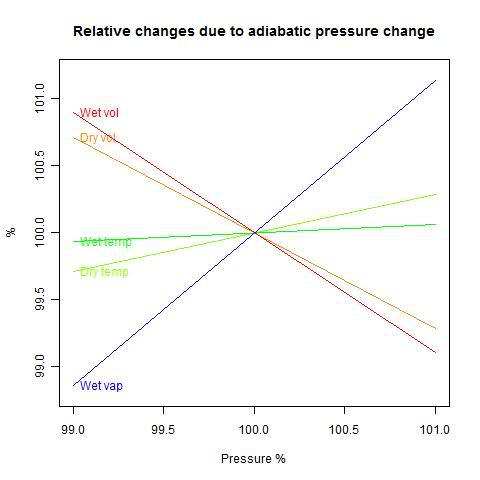First observation - wet DT/DP is much smaller. Condensation is stabilizing the temperature, as you'd expect. And a 1% drop in P causes 0.916 1.14% of water vapor to precipitate.

But the answer I was looking for is the third. A 1% drop in P increases the volume by 0.861 0.896% - more than it does for dry air (0.714%). The condensation causes the volume to expand relative to non-condensing air.

#### Interpretation

This study was just of air in a confined space. But it can be applied to saturated air rising in parcels in the atmosphere. The dry lapse rate is the temperature gradient which is neutral for air moving without condensation (more here). So the moist air is cooling more slowly than the lapse rate as it rises, and is expanding relative to air on its level.

#### Update - more algebra

Equations 4-6 can be rewritten in even fewer non-dimensional parameters. I'll take the ratio cv/R = 5/2.

Then with b=L/(R*T), and c=(L*nw)/(n*R*T)

7)      2.5 DT + c Dnw + DV = 0
8)      - DT - (c/b) Dnw + DP + DV= 0
9)      -b DT + Dnw + DP = 0

Eqns 7-9 are linear homogeneous equations in the four variables: (DT, Dnw, DP, DV) The answer can be expressed in terms of a vector a=( a1,a2,a3,a4 ) and an arbitrary parameter h:
DT=a1h,  Dnw=a2h,  DP=a3h,  DV=a4h.

Finding a is a matter of linear algebra (school algebra or determinants). I'll just state the result and verify:

a1 = 1 + c + c/b,  a2 = b - 3.5,  a3 = 3.5+c+b*c,  a4 = -(2.5+b*c+2.5*c/b-c)

Substitute in (7) with h=1 (h will cancel):
2.5*a1 + c * a2  + a4  =  2.5+2.5*c+2.5*c/b + c * b-3.5*c - 2.5 - b*c+-2.5*c/b+c  =  0
And (8): -a1 -(c/b)* a2 + a3 + a4  =  1- c-c/b  - c+3.5*c/b + 3.5 + c + b*c - 2.5 - b*c-2.5*c/b+c  =  0
And (9): -b*a1+ a2  =  -b-c-b*c + b- 3.5 + 3.5+c+b*c = 0

You can use this result to get any desired derivative, eg DV/DP = a4/a3
Remember again that D is proportional, so dV/dP = (V/P) a4/a3.

Update. I see that Eq 3.74 of Rodrigo Caballero's PhysMet lecture notes gives a version of DT/DP which is equivalent to (1+c)/(3.5+bc). He says this is an approximation (3.72). I think that is equivalent to omitting the last term in my Eq 2, which would indeed give this expression. However, I believe the inclusion of that term is more accurate.

Appendix - R code
V=25
T=300
N=1000
Nw=36   #0
P=100000
R=8.3
Cv=R*2.5
L=45000

LRT=L/R/T
m=matrix(c(Cv/R,-1,-LRT,  LRT*Nw/N, -Nw/N,1, 1,1,0, 0,1,1),3,4);

M=m[,c(1,2,4)]; rh= -m[,3];
ddV=solve(M,rh);    # D/DV volume derivatives

M=m[,c(1,2,3)]; rh= -m[,4];
ddP=solve(M,rh);    # D/DP pressure derivatives

print(ddV);  print(ddP);

## Wednesday, October 20, 2010

### ## A ramble on GCMs Navier-Stokes equations.

This post is an outgrowth of a discussion at the Air Vent on GCM's and condensation. It's a kind of response to comment claims that GCN's don't allow for inversion, or volume change during condensation etc. I found myself saying often that it all comes out in the solution of the Navier-Stokes equations - you don't have to do something special.

It's often said that the N-S equations are at the heart of GCM's, but if you look at a set of equations actually solved, as in this set from CAM3, it isn't obvious. And it's made less obvious by the funny coordinates they use.

But it's true, and here I'll try to set out the reasons.

#### The Navier Stokes equations

These go back to Navier in 1822, although his physics was eccentric, based on a stretched version of recent atomic theory. GG Stokes in about 1845 gave a definitive derivation and solved most of the special cases that could be done theoretically. In the mean time, Poisson and Saint-Venant also made big contributions. In fact, what I'll be talking about mainly goes back to Euler.

I'll write out a version for slightly compressible fluids. An abstract form is:
 ρDv/Dt = -∇.τ Momentum equation Dρ/Dt = -∇.(ρv) Mass conservation or continuity ρ-ρ0=(P-P0)c-2 Constitutive Relation
Update - I've added a P0 to that last equation (I'm used to thinking of ambient pressure as 0, but of course in the atmosphere that won't do).  At Lucia's suggestion I've used the more orthodox term constitutive relation (not eqn of state). I'll also mention that ρ is density, v velocity, P pressure and t time.
D/Dt represents a total or advective derivative - in a frame moving with the fluid. ∇ is a vector derivative operator (∂/∂x,∂/∂y,∂/∂z), and τ is a stress tensor. Don't worry about what that means if it's unfamiliar - it's about to disappear.

I've chosen this "abstract" style to show the structure. The momentum equation is just Newton's second Law -   ma = F . Continuity just says that if mass is moved by advection (being carried with the flow), the density will change. And this constitutive relation just says that to change the density from a standard (local hydrostatic) value, you have to apply a pressure P, and c is the speed of sound. You could have other constitutive relations for different materials.

The stress tensor could be quite complex, but for now, let's just say there's a pressure P, gravity g, and a viscous stress (coef μ). Atmospheric flows are always turbulent, and turbulence models can work by modifying the viscosity. But I mainly want to talk about the pressure-velocity interaction, so I'll just keep a viscous term as an example of the other things that could come in.

OK, so now we have:

 ρDv/Dt = -∇P + ρg + ∇.(μ∇v) Momentum equation DP/Dt = -ρc2∇.(v) Mass conservation or continuity

#### Time Scales and numerical solution

Suppose you wanted to solve these numerically. You'll have the space divided up into regions, with the field variables (P, v etc) represented on nodes - maybe the corner points. You'll have linear expressions for the space derivatives in terms of nodal values.

So a naive approach is to say - suppose you calculate the right hand sides at a point in time. just calculate the rates of change of v and P, and assume that holds for a timestep. Update and proceed. That's called an explicit method (forward Euler).

But it won't work, except for very small time steps. The reason is that, while you've got starting information within your little regions, what's going on in one region can affect others during that step. You're limited by the fastest process that can propagate physical effects. And that is the speed of sound, which is the way pressure is propagated.

The reason is that buried in the N-S equations is the acoustic wave equation:
 ρdv/dt = ∇P dP/dt = -ρc2∇.v+...
or
 c-2d2 P/dt2 = ∇2P+R1
or
 c-2d2v/dt2 = c2∇2v +R2
I've replaced D by d, because only fast processes are retained, and the difference between D and d is an advective term, which is also slow relative to sound speed. R1 and R2 denote the slow terms.

By small time steps, I mean small enough so that a sound wave will only move part way across one of the small regions. In GCM's, the vertical divisions can be just a few hundred metres. That's a second or so of sound time. That would be ridiculously short for GCM modelling, where steps of about half an hour are common. Incidentally, these are constrained by the speed of other "weather-like" processes.

#### Coupling and semi-implicit solution

The problem is that with the explicit method, we have to resolve the acoustic oscillations, which are not of interest.

Looking at the pressure wave equation, we can say that, since c is large, only acoustic solutions will have a significant left side. For the solutions we actually want, that can be dropped. Then we get

2P  = - R1

wher again R1 is a collection of slow terms. This is what is called the Pressure Poisson equation. Once solved, the pressure can be used to get the velocity by going back to the momentum equation.

The essential thing here is taking the pressure and velocity equations together - coupling. Everything else can be held constant during the time step (though you can also do better with staggered time steps etc - there's lots of extra art not relevant here).

So now the time step can be extended until the next fastest process will mean that the computational cell cannot be regarded as (sufficiently) isolated during the timestep. Since that process is generally, unlike sound, one of the processes you do want to resolve, that's basically it.

#### Summary

So that's why the Navier-Stokes equations are the core. They can be seen as an engine that matches pressure and velocity, using sound implicitly, but not requiring sound-limited timesteps. Once you have that sorted out in a time-step, all the other physical processes can be updated in good time.

#### Heretical afterthought - for practitioners

Actually, implicit solution is not as rosy as it is often painted. You can take long time-steps, but you have to solve a big matrix of equations to do it, instead of just calculating new values at each node. You take advantage of the fact that each node only affects a few neighbors, which gives a sparse matrix. So for big systems you use an iterative solver.

The gloomy fact is that the iterative solver, in effect, is an accelerated version of the original explicit solution. And using something like conjugate gradients, the acceleration is significant, but not super. Preconditioning helps, as does a process called multigrid. But the fact remains that, as you extend the timestep, the matrix becomes more ill-conditioned (an analyst's curse word), and you end up with a number of iterations rather related to the number of explicit steps you would have had to perform.

I might say more about preconditioning in a future post.

## Thursday, October 14, 2010

### ## Wahl & Ammann proxy calculations

I had been quietly plodding through Wahl and Ammann 2007. This is the paper that re-did the MBH98/99 proxy calculations using conventionally centred principal components analysis and a modified number of PC's. It's relevant to recent discussions of the Wegman report.

Some original code and data used in the paper is available at Nychka's site at NCAR. So I downloaded it. It's elegant in R. Just 11 lines of actual calculation to get from the proxy data to a set of proxy eigenvectors, with decentered scaling. And then, of course, R does the regular PCA for comparison in one line.

Then Ron Broberg put up a very helpful post drawing attention to a discussion by Dr Richard Smith of a recent paper by Li, Nychka and Ammann. As Ron says, it's well worth reading (as is Ron's post). I've only just started.

#### The R code

To get me going, I re-commented Ammann's code, to help my understanding. I'll show it, with the Figures, below the jump.

So the next stage is for me to recheck the things that are commonly said about the various proxies, and how they are reweighted. Later it might be possible to adapt the code for the Mann08 (Tiljander etc) analysis.

Here's Ammann's code for the decentred PCA. My comments have a double ##:

## scan a big file of proxy data - 70 proxies, 581 years from 1400:1980
## North America
T<-scan("2004GL021750-NOAMER.1400.txt",skip=1)
T<-matrix(T,nrow=581,ncol=72,byrow=TRUE)
tree<-T[1:581,3:72]
## tree is the matrix of proxy data over 1:581 years (1400:1980) - 70 proxies

tt<- c(1400:1980)

# MBH- uncentered PCs  GRL Data, Caspar Ammann's code
# =======
## This part rescales tree according to the mean and sd to get xstd
m.tree<-apply(tree[503:581,],2,mean)
s.tree<-apply(tree[503:581,],2,sd)
xstd<-scale(tree,center=m.tree,scale=s.tree)
## regress 1902:1980 columns of xstd against years in calibration period
fm<-lm(xstd[503:581,]~c(1:nrow(xstd[503:581,]))) #fit linear model to calibrati\$
## fm(\$residuals) is the set (79x70) of residuals
## sdprox(1:70) is the sd for each proxy over the 79 years
sdprox<-sd(fm\$residuals)                         #get residuals
## so now the xstd are rescaled relative to the calib period.
## This creates the famous MBH98 decentering (note center=F)
mxstd<-scale(xstd,center=FALSE,scale=sdprox)     #standardize by residual stddev
## Now do the svd decomposition on mxstd  = u * d * v
w<-svd(mxstd)                                    #SVD
## w\$u is the 581x70 matrix of ordered eigenvectors
## w\$d are the eivals =  164.3 83.1 58.9 50 45.6 ...
PCs<-w\$u[,1:5]                                   #Retain only first 5 PCs for c\$
## At this stage, "retain" is just the number of PC's that we calculate
m.pc<-apply(PCs[503:581,],2,mean)
s.pc<-apply(PCs[503:581,],2,sd)
PCs<-scale(PCs,center=m.pc,scale=s.pc)
## Now each PC is rescaled to mean=0, sd=1, again on 1902:1980

## Now just plot - fig1() and fig2() are plot routines which also call princomp().
source("fig1.R") # only need to source these fucntion once if they
source("fig2.R") # are saved in your work space.

## I changed the output from pdf to jpg for html posting
jpeg("fig1.jpg", width=900, height=800)
fig1()
dev.off()

jpeg("fig2.jpg", width=900, height=600)
fig2()
dev.off()

Here's the routine for fig1(). Note the one-line calcs for the regular PCA:

`fig1` <-
function(){

par( mfrow=c(3,1), oma=c(6,0,0,0))
par( mar=c(0,5,2,2))

## This does the whole PCA by the covariance method (cor=F is the default)
## It returns the "scores" - ie the first 5 (here) principal components
PCs.3<- princomp(tree)\$scores[,1:5]

## This does the PCA by the correlation method
PCs.2<- princomp( tree, cor=TRUE)\$scores[,1:5]

## tt is 1400:1980. PCs[,1] is first PC. The scale() seems redundant
## but isn't, because it was previously scaled to the calib period.
plot( tt,  scale(PCs[,1]), type="l", lty=1, xaxt="n", ylab="Scaled PC1")
abline(h=0,col="blue")
title("MBH centering and scaling in instrumental period",adj=0)

plot( tt,  -1*scale(PCs.3[,1]), type="l", lty=1,xaxt="n", ylab="Scaled PC1" )
abline(h=0,col="blue")

plot( tt,  scale(PCs.2[,1]),

type="l", lty=1, xaxt="n", ylab="Scaled PC1")
abline( h=0, col="blue")

par( oma=c(0,0,0,0), mar=c(5,5,2,2))
axis( 1)
mtext( side=1, line=3, text="Years")

}

Here's the resulting plot:Here's the routine for fig2():

`fig2` <-
function(){

par( mfrow=c(2,1), oma=c(5,0,0,0))
par( mar=c(0,5,3,2))

## as for fig1()
PCs.3<- princomp(tree)\$scores[,1:5]

PCs.2<- princomp( tree, cor=TRUE)\$scores[,1:5]

## Plot the decentred MBH PC1
plot( tt,  scale(PCs[,1]),
type="l", lty=1, xaxt="n", ylab="Scaled PC1")
abline( h=0, col="blue")
title("First five covariance PCs projected (green)
onto MBH centered and scaled PC (black)",adj=0)

## Regress the covariance PCs against decentred
lm( scale(PCs[,1]) ~ PCs.3[,1:5] ) -> out
## Plot the resulting fitted curve
lines( tt, predict(out), col="green", lwd=2)
lines( tt, scale(PCs[,1])) ## ?? this just seems to replot the MBH PC1

## Same, but done for the correlation method
plot( tt,  scale(PCs[,1]),
type="l", lty=1, xaxt="n", ylab="Scaled PC1")
abline( h=0, col="blue")
title("First five correlation PCs projected (green)
onto MBH centered and scaled PC (black)",adj=0)

lm( scale(PCs[,1]) ~ PCs.2[,1:5] ) -> out
lines( tt, predict(out), col="green", lwd=2)
lines( tt, scale(PCs[,1]))

par( oma=c(0,0,0,0), mar=c(5,5,2,2))
axis( 1)
mtext(side=1, line=3, text="Years")

}

Here's the plot: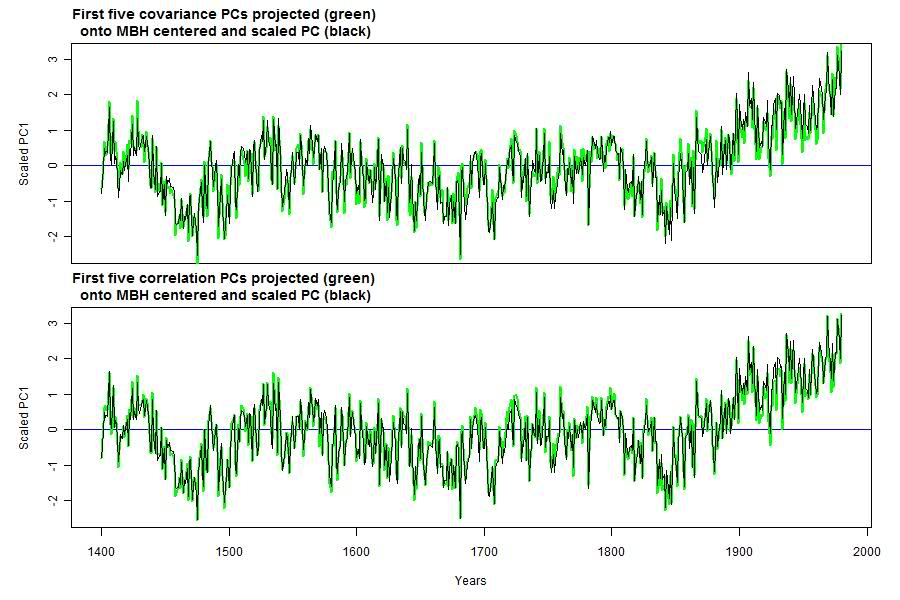I'll comment more on the results in the next post, and also look in more detail at the various PC's.

## Wednesday, October 6, 2010

### ## Can downwelling infrared warm the ocean?

Science of Doom has raised this question, which does crop up more frequently than it ought. Doug Hoyt, who should know better, has given it impetus.

It goes like this. Water is opaque to thermal IR, and not a good conductor. IR penetrates only a few microns. Therefore heat from incident IR from above must ...

be all converted to latent heat

or, be reflected, or ...

Land is also opaque to IR, but the idea that IR can't warm it doesn't seem to have the same attraction

Some thoughts below the jump.

#### Can the ocean emit thermal IR?

This is an equally good question. But it certainly does. In fact, that's how satellites measure SST (skin temperature).

A large part of the insolation that penetrates the sea, to a depth of several meters, is later radiated in this way. It's a big part of the thermal balance. Somehow, that heat is conveyed to the surface, and is emitted by the surface layer.

That pathway is a mix of turbulent advection, conduction in the top few mm, and radiation in the top microns. All those mechanisms are bi-directional. If heat can be emitted, it can be absorbed.

#### Heat balance

On average, the surface loses heat, by evaporation and radiation (and some conduction to air). Incoming IR does not generally need to be absorbed. It simply offsets some of the emission.

There is some diurnal effect, so that there depth intervals where the heat flow during the day is downward.

I've said that heat can be transported to and across the interface. But of course there is some resistance, and this produces a temperature gradient. The temperature differences are noticeable, but not huge. Dr Piotr Flatau has posted some results on Wikipedia:Note the nearly logarithmic depth scale. The top 10 microns, in which there is nett daytime emission, shows a drop of a bit less than 1°C. There is another drop of about half a degree in the conductive layer. Then the gradient changes, reflecting presumably the upflux of heat from the more rapidly absorbed solar wavelengths. The gradient then diminishes as the source fades, and the turbulent eddies grow in size, increasing turbulent advection.

At night, the gradient becomes monotonic, with the advected flux uniformly upward. But again the temperature difference is less than a degree:#### Conclusion

In a very technical sense, the sea is heated by sunlight rather than downwelling IR, as is the land. That's just trivial arithmetic - the sun is the heat source. And the upward IR flux generally exceeds the downward. But downwelling IR does add joules to the sea just as effectively as SW - there is no blocking thermal resistance.

## Saturday, October 2, 2010

### ## An entropy budget for the Earth

This post follows up some ideas in comments to the Science of Doom post on 2LoT. Whenever you calculate a heat flux, and you know the temperature, you can calculate the entropy changes. Tracking entropy is different from tracking energy flux, in that entropy is not conserved, but will be created whenever there is irreversible heat transfer. However, it is conserved in reversible processes, and can never decrease globally, although it can be transferred to somewhere else. And as with heat, for Earth steady state requires that entropy that enters or is created, must leave.

So I have the idea of doing an entropy budget, analogous to Trenberth's famous energy budget for the Earth. It's a bit more complicated though, so this post will look at a simplified Earth.

#### Entropy calculations

Whenever heat Q Joules is added to a region at constant temperature T, the entropy increases by Q/T J/K. If Q is negative (heat is lost), then so is entropy (to that region). If T is not constant, you have to integrate, but the idea is the same.

By the Second Law, if the heat flows from one region to another in an otherwise isolated system, the second must be the cooler, and it will gain more entropy than the first lost.

In these examples, we'll be dealing with fluxes, so Q is replaced by F, the flux in J/s/m^2, or W/m^2, and the result, S, is an entropy flux in W/K/m^2.

#### Entropy significance

The entropy associated with a heat flux is associated with its capacity to do work. In fact, a Helmholtz (or Gibbs - same since we're not considering PV work here) free energy can be defined:
A = F - TS
remembering that A, F and S are flux densities. A is a measure of the power that could be delivered as mechanical work from the heat flux F if discharged to a heat sink at temperature T.

This can be used as a more rigorous definition of the entropy flux associated with an E/M flux:
S=(F-A)/T,
where again, A is the mechanical power that could theoretically be generated by using the flux F with heat sink at T.

As a heat flux passes to cooler regions, it loses some capacity to do further work. However, in that transfer it could have done work if a heat engine mechanism existed (equivalent to the capacity it had subsequently  lost). So when you see entropy increasing, it means that where that happened, there was a capacity to generate weather effects, etc.

#### Snowball Earth

 I'll introduce a symbol for a heat/entropy flux. It's an arrow with three numbers. In the middle, in red, is the heat flux. At the tail, in black, is the entropy flux associated with the origin of the flux, and at the head is the entropy transferred to the heat sink. So here you see the basic calc for average fluxes for snowball Earth, with a clear atmosphere with no GHG. Incoming solar is 342 W/m2, but 107 W/m2 is reflected (assuming Earth's true albedo still applies), so at balance 235 W/m2 is emitted directly to space as IR. The surface temperature required to do that is 255K. Entropy (820 W/K/m2) is created when SW is thermalised at the surface. This is an irreversible process. The exchange could have driven a heat engine, but didn't. No further irreversible process occurs, and the entropy is exported to space unchanged.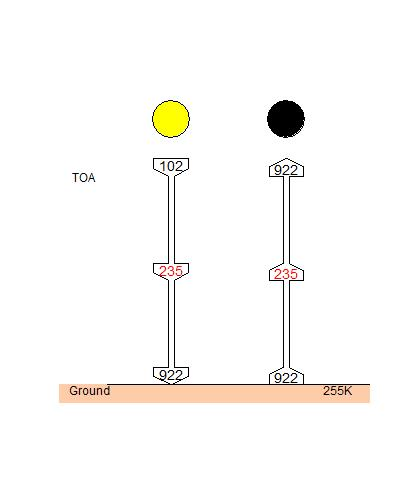In this calculation the incoming temperature was assigned a value of 2310K. The apparent emission temperature from the sun is 5700K, so why the reduction?

It is a subtle point, but while incoming SW has the theoretical ability to heat a black body to 5700K (with concentrating mirrors etc), at that temperature all the energy is radiated back to space, and cannot be used to perform work. There is a discussion here. The optimum temperature for conversion of solar energy to work is 2310K.

The effect of the difference is slight - on either count, incoming entropy is small.

#### Earth with GHG shell

 GHG's in the atmosphere absorb and emit radiation, some of which returns to the surface, and some of which exits to space. Most of the 235 W/m2 received by the Earth's surface is returned to space emitted from GHG's at high altitude. The resulting transfers create entropy in a rather distributed way. However much of the effect is captured by a simplified model in which GHG's are confined to a narrow band near TOA, at 225K. This band has an atmospheric window, 40 W/m2. As with the Snowball Earth, that flux gains no further entropy. However, the proportion absorbed at the shell does yield an entropy change. Some returns to the warmer surface, with an entropy decrease. And some is emitted to space, with entropy loss to the Earth (and gain by space). The total export of entropy is 1006 W/K/m2.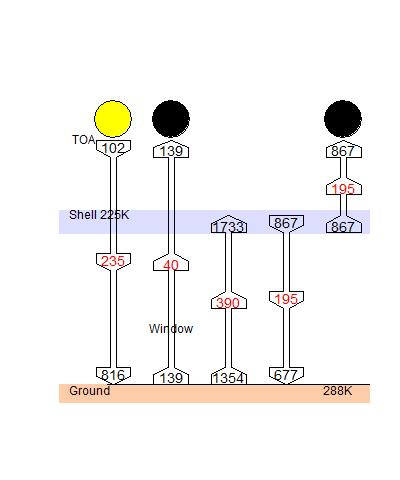The entropy decrease has been the subject of argument at SoD. However, from a budget point of view, the up arrow from ground to shell, and the down arrow, could be merged, with a tail of 667 W/K/m2 and a head of 867, and a net entropy gain of 220 W/K/m2. This is what counts for 2LoT - the nett change in entropy, which is positive.

#### The Greenhouse Effect and entropy

Comparing with and without GHG:
• With GHG there is less entropy created by incoming SW at the warmer surface - 714 W/K/m2 vs 820.
• With GHG the total export of entropy is higher - 1006 W/K/m2 vs 922.
• The entropy gain from ground to shell of 220 W/K/m2 can be associated with heat engine effects in the atmosphere, or with life processes etc. The colder shell is necessary to export the difference.

#### Realistic?

Yes, in terms of budgetting. The shell artifice misrepresents the apparent temperature of the downwelling IR (at 225K) - it's much higher. The 190 W/K/m2 entropy reduction does not occur at the surface, but distributed through the atmosphere. Likewise, the upwelling stream entropy increase is likewise distributed, But this does not affect the budget.

And yes, I've ignored clouds.

## Beta version of GHCN v3

h/t CCE (at Whiteboard).
I've only recently read CCE's comment - I've downloaded v3 from here. It's late where I am, so this is very much a first impression.

The README file is helpful. The inventory file has a new layout, so TempLS will need some changes to read it. It seems to have basically the same data, though, about the same 7280 stations as v2.

The data file also seems to have much the same actual data. But interspersed are a number of codes. There is a measurement code and a quality code, but not yet used, except for USHCN. Then there is a source code (saying where the data comes from).

So there's work to do to get TempLS to read it. It's not clear that this beta version has data changes that will affect the results. But we'll see.
Update below the jump:

Update: I found this useful set of slides from a talk by Dr Karl in May 2010. It has several plots based on V3. I had been planning to do a v2/v3 comparison, but there's one there: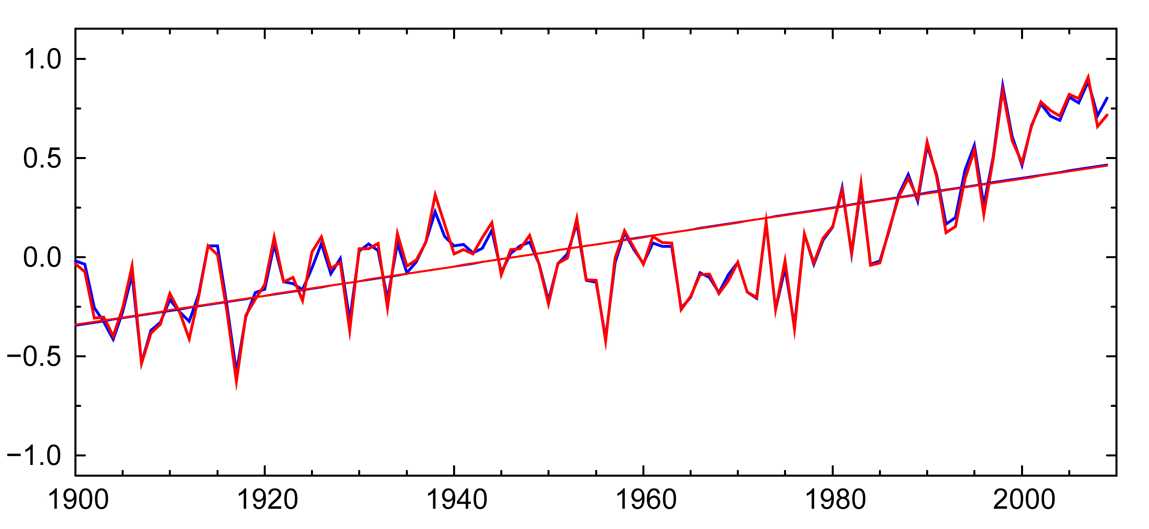Slide 21 - units C, blue v2, red v3

There's also a more thorough examination of v3 from Zeke, referenced in his comment. He has also done the v2/v3 comparison, as well as a station count (V3 has more readings, but no new stations), and a look at adjustments.

Mosh says there that the main diff with the dataset is that they have eliminated many (all?) duplicates. Indeed, there are 443933 lines in the file, vs 597182 in v2.mean.

## Monday, September 13, 2010

### ## GISS for August 0.53C, same as July

GISS is rather early this month - seems to be even before MSU-RSS. It's the one index that had been falling rather rapidly, so no change is a change.

The monthly land/ocean global plots below are from Moyhu's monitoring page.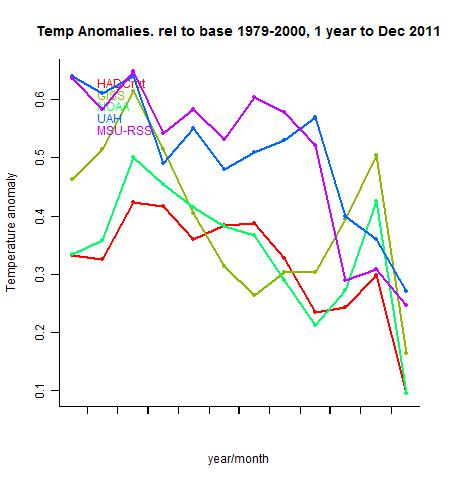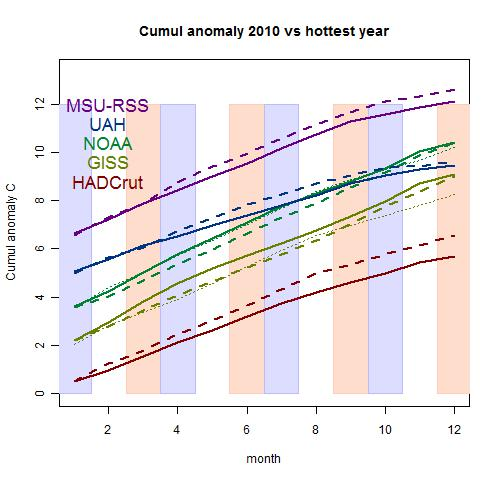Note the change of base, to put all indices on an equal footing.

## Friday, September 3, 2010

### ## A very different gridding method - TempLS

 Station-based monthly global temperature indices use gridding at some stage, and usually the grid cells follow some regular rule, like a lat/lon square. This causes some problems: Minor - cells are unevenly populated with stations. Major - some cells have no data in some months These can be avoided with the use of irregular grid cells, which can be smaller when stations are dense, and can be formed with a requirement that they always have at least one data point.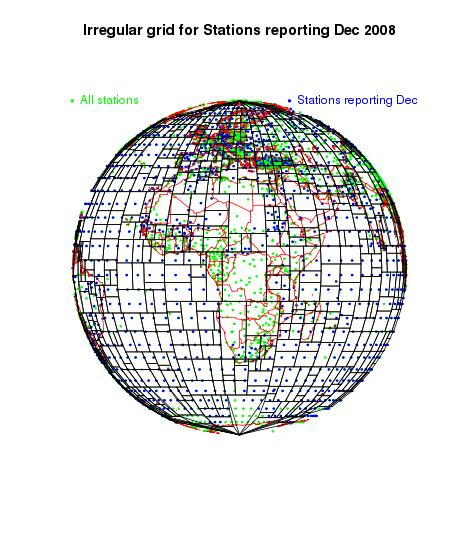There are needs here which make well-known unstructured meshes like Voronoi unsuitable. This post describes one based on binary trees.

#### The purpose of gridding

The naive way to get a world average temperature anomaly is just to add results for all the stations. But it gives wrong results when stations are concentrated in certain regions. And it underrates SST.

The usual way is to create a regular grid, and in some way average the readings in each cell. Then an area-weighted mean of those cell averages is the result.

This gives a fairly balanced result, and has the same effect as numerical surface integration, with each grid a surface element, and the integrand represented by the cell average.

TempLS does something which sounds different, but is equivalent. It forms a weighting based on inverse cell density, expressed in each cell as cell area divided by the number of data points in the cell.

Another way of seeing that is to imagine that the cell was divided into equal areas, one containing each cell. Then the weights would be just those areas.

#### Empty cells

Empty cells are usually just omitted. But if the temperature anomaly averaged over just the cells with data is presented as the global average, necessarily some assumption is implied as to their values. If you assumed that each missing cell had a value equal to the average of the cells with data, then including them would give the same answer. So this can be taken to be the missing assumption.

Is it a good one? Not very. Imagine you were averaging real temperature and had a lot of arctic cells empty. Imputing world average temperatures to them is clearly not good. With anomalies the effect is more subtle, but can be real. The arctic has been warming more rapidly than elsewhere, so missing cells will reduce the rate of apparent warming. This is said to be a difference between Hadcrut, which just leaves out the cells, and GISS, which attempts some extrapolation, and gets a higher global trend. GISS is sometimes criticised for that, but it's the right thing to do. An extrapolated neighbor value is a better estimate than the global average, which is the alternative. Whatever you do involves some estimate.

#### Irregular cells and subdivision

Seen as a surface integral approx, there is no need for the cells to be regular in any way. Any subdivision scheme that ascribed an area to each data point, with the areas adding to the total surface, would do. The areas should be close to the data points, but needn't even strictly contain them.

Schemes like Voronoi tesselation are used for solving differential equations. They have good geometric properties for that. But here there is a particular issue. There are many months of data, and stations drop in and out of reporting. It's laborious to produce a new mesh for each month, and Voronoi-type tesselations can't be easily adjusted.

#### Binary tree schemes

I've developed schemes based on rectangle splitting, which lead to a binary tree. The 360x180 lon/lat rectangle is divided along the mean longitude of stations. Then the rectangle with longest side is divided again, along the lat or lon which is the mean for stations in that rectangle. And so on, but declining to divide when too few stations would remain in one of the fragments. "Too few" means 2 at the moment, but could be refined according to the length of observations of the stations. There's also a minimum area limit.

That's done at the start. But as we go through month by month, some of those rectangles are going to have no data. That's where the binary tree that was formed by the subdivision comes in. It is the record of the divisions, and they can be undone. The empty cell is combined with the neighbor from which it was most recently split. And, if necessary, up the tree, until an expanded cell with data is found.

#### Weights

In binary tree terminology, the final set of rectangles after division are the leaves, and adding a notional requirement that for each month each cell must contain data leads to some pruning of the tree to create a new set of leaves, each being a rectangle with at least one data point. Then the inverse density estimate is formed as before - the cell area divided by the number of data. Those are the weights for that month for each cell.

#### Does it work?

Yes. I've produced a version of TempLS V2 with this kind of meshing. A picture of the adapted mesh for Dec 2008, GHCN, is above. I'll show more.

The mesh manipulations, month to month, take time. I've been able to reduce the run time for a Land/Sea GHCN run to well under a minute, but the irregular mesh doubles that. I'm hoping to improve by reducing the number of tree pruning ops needed.

#### Results

I did a run from 1979 to 2008. Here are the trends using standard TempLS and the irregular grid version. At this stage, I just want to see that the results are sensible.
 Trend Reg_grid Irreg_grid Number 9263 9263 1979-2008 0.1636 0.1584 Trend_se 0.02007 0.02119

And here is a superimposed plot of annual temperatures: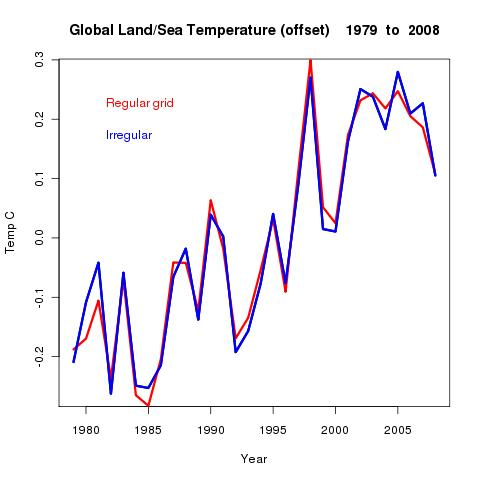#### So what was achieved?

Not much yet. The last plot just shows that the irregular mesh gives similar results - nothing yet to show they are better. I may do a comparison with GISS etc, but don't expect to see a clear advantage.

Where I do expect to see an advantage is with an exercise like the just 60 stations. I think in retrospect that that study was hampered by empty cells. In fact, almost every station had a cell to itself, which meant they were all equally weighted, even though they were not evenly distributed.

Work is needed to adapt the method to region calculations. If the region can be snugly contained in a rectangle, that's fine. But the surrounding space will, by default, be included, which will overweight some elements.

One virtue of the method is that it has something of the effect of a land mask. With a regular grid, coastal cells often contain several land stations and a lot of sea, and in effect, the sea has the land temperature attributed to it. The irregular grid will confine those coastal stations in much smaller cells.

#### More mesh views

Finally, here are some more views of that mesh used for December 2008: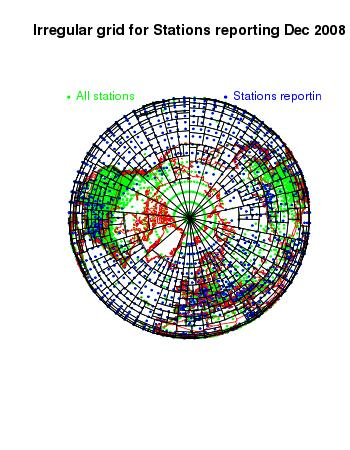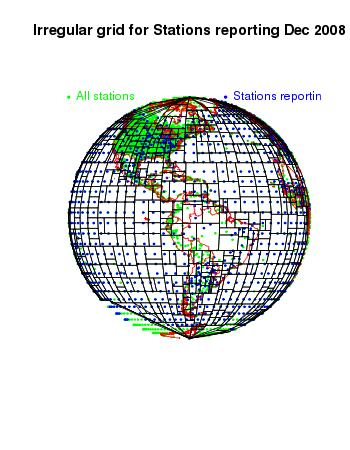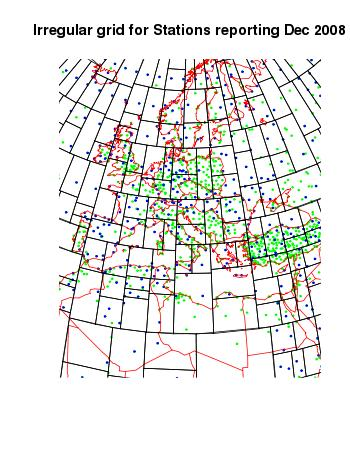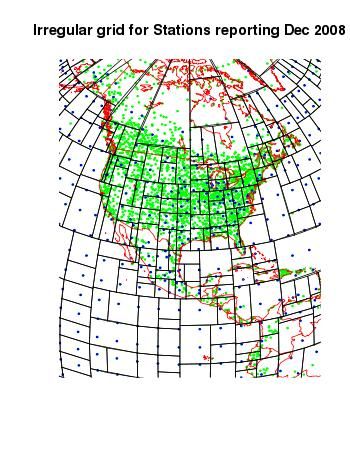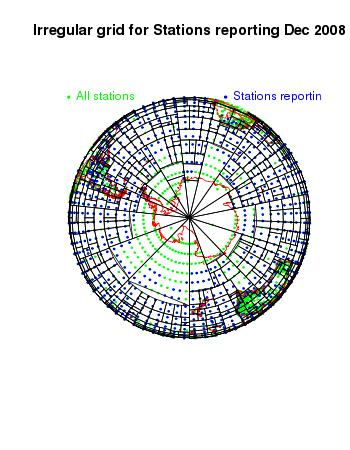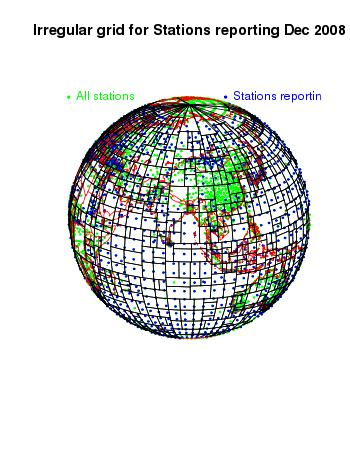The mesh enlargement in the Antarctic seems to overshoot somewhat - it makes large elements with quite a lot of nodes. This should be improveable. There are some big elements in N Canada - this was the period when few stations there were reporting.

## The Math basis of TempLS Ver 2

For Version 1 of TempLS I posted a PDF guide, TempLS.pdf, to the mathematical basis. It's a useful starting point here.

But this time I'm going to try to do it in HTML below. Colors help.

For more Version 2 information:

• Index of posts by category
• TempLS Version 2 Release
• Ver 2 - Regional spatial variation.
• Spatial Temperature distributions in TempLS V2
• Plotting spatial trends in TempLS

• #### Notation

I need to use a summation convention. I'm referring to various matrices etc as just indexed sets, and when they are multiplied together, every index that appears twice is understood to be summed over the range of that index. It's called a dummy index, because it doesn't matter what symbol you use (as long as it's not used for something else). Once the summation is done, that index doesn't connect with anything else.

I'll be talking about weighted least squares, and an exception is needed for the weight function. It's indices are sometimes put in brackets, which means they should not be counted in this pairing. The index still varies with any summation that is going on.

The reason for this is that summation signs are difficult in HTML. But also it gets easier with colors. I'll color indices subject to summation blue, standalone indices red, and exception indices lime green.

So multiplying two matrices A and B (C=A*B) would be represented:
Cik  =  Aij Bjk

#### The model

We have, on the GHCN and similar sets, a number of stations (s), with temperature readings (x) by year (y) and month (m). m varies 1:12. The model is that x can be represented by a local temperature L, with a value for each station and month (seasonal average), and a global temperature G depending on year alone.

xsmy  ~  Lsm  +  Gy
It's useful to maintain a convention that every term has to include every red index. So I'll introduce an identity Iy, for which every component is 1, and rewrite the model as
xsmy  ~  IyLsm  +  IsmGy

#### Weighted least squares.

The L and G are parameters to be fitted. We minimise a weighted squares expression for the residuals. The weight function w has two important roles.
• It is zero for missing values
• It needs to ensure that stations make "fair" contributions to the sum, so that regions with many stations aren't over-represented. This means that the sum with weights should be like a numerical integral over the surface. w should be inversely proportional to station density.
So we want to minimise w(smy)(xsmy - IyLsm - IsmGy)2

#### Differentiation

This is done by differentiating this expression wrt each parameter component. In the summation convention, when you differentiate wrt a paired index, it disappears. The remaining index goes from blue to red. The original scalar SS becomes a system of equations, as the red indices indicate:
 Minimise: w(smy)(xsmy - IyLsm - IsmGy)2 Differentiate wrt L: w(smy)Iy(xsmy - IyLsm - IsmGy) = 0 Differentiate wrt G: w(smy)Ism(xsmy - IyLsm - IsmGy) = 0

The identity operations have been useful, but can now be rationalised. The equations become:

 Differentiate wrt L: w(sm)y IyLsm  +  wsmy Gy  =  w(sm)y xsmy Differentiate wrt G: wsmy Lsm  +  wsm(y) IsmGy  =  wsm(y) xsmy

#### Equations to be solved

These are the equations to be solved for L and G. It can be seen as a block system:
A*L + B*G  = X1
BT*L + C*G  = X2
combining s and m as one index, and where T signifies transpose. Examining the various terms, one sees that A and C are diagonal matrices, so the overall system is symmetric.

The first equation can be used to eliminate L, so
(C -  BTA-1B)*G = X2  -  BTA-1X1
This is not a large system to solve - G has of order 100 components (years). But the multiplications to create it are time-consuming. The alternative offered by TempLS is to use the conjugate gradient method on the whole system. This turns out to be fast and reliable.

There is a rank deficiency in that a constant could be added to L and subtracted from G. This is resolved by forcing the first component of G to be zero. Later this can be adjusted to set the base period for G as an anomaly. The same issue arises with spatial models below.

#### Spatial dependence

That is what was done in Ver 1. In version 2, it is called model 1.The weight function w was estimated using a grid to get the station density locally.

But we could try to put more parameters into G. In particular, we could try to let it vary spatially, and this is what is new in V2.

We need a set of orthogonal functions, expressed as a matrix Psk, being a value at each station, where k indexes the functions. Actually, the functions needn't be strictly orthogonal, but should express the range of possible spatial variation.

The continuous function that this produces is Pk(lat,lon)Hk, where H is a set of coefficients to be found.

Then it's simpler if the dependence of G on years is fixed in advance. A model expressing these dependences is:
xsmy ~ IyLsm +  ImJyPskHk
For J, there are two forms used:
• J=y, yr0≤y≤yr1 gives a trend in C/yr between those years. This is model 2
• J=1, yr0≤y≤yr1 gives an average C between those years. In Ver 2.0, this is model 3, and is restricted to a single year.

#### Differentiation

This time the sum of squares  of residuals is differentiated wrt L and H:
 Minimise: w(smy)(xsmy - IyLsm -  ImJyPskHk)2 Differentiate wrt L: w(smy)Iy(xsmy -  IyLsm -  ImJyPskHk) = 0 Differentiate wrt H: w(smy) ImJy Psk (xsmy -  IyLsm -  ImJyPsjHj ) = 0

Again it can be re-expressed as a symmetric system:
 Differentiate wrt L: w(sm)y IyLsm  +  w(s)my JyPskHk  =  w(sm)y xsmy Differentiate wrt H: w(s)my Jy Psk Lsm  +  w(smy) ImIm JyJy Psk Psj Hj  =  w(s)m(y) Jy Psk xsmy

#### Solving

Solving is by the same elimination method - the multiplier of L in the first equation is again a diagonal matrix. The conjugate gradient method is less attractive here.

Spatial methods can be used over the whole globe or over sub-regions. There is a tension between the resolution you would like to get by using many orthogonal functions, and the information available. If there are too few stations for the resolution, the final matrix will be ill-conditioned, and spurious results will occur.

TempLS deals with this by doing a diagonalization of that final symmetric matrix, and inverts a subsystem based on the larger eigenvalues - a kind of PCA. The user can specify where the cutoff occurs, using a variable eigcut. This should be a small number, between 0 and 1, but closer to 0. I often use 0.01 - higher values are more conservative. A strategy is to try different values until the results do not depend on your choice.

#### Orthogonal functions

The types currently available are
• Type 1 - spherical harmonics, for the whole sphere
• Type 0 - trig functions (of lat-lon) for use on approximately rectangular regions
The number num_orthogs in the user file gives the approximate resolution (in half-periods)in one dimension. The total number of functions generated is approximately the square of this. Values in the range 8-12 are common - maybe up to 16 on the globe. Remember that it will generally be over-ridden by the eigcut facility - ie if you set num_orthogs too high, you'll just do some extra work for the same result, while if you set it low, you won't get the resolution that you could.

## Saturday, August 14, 2010

### ## Hottest year? 2010?

A frivolous topic, to be sure, but it will probably be discussed in coming months. Will 2010 set any kind of hottest year record? It doesn't look particularly likely, but I'm sure people will speculate. So I've produced a tracking plot. It shows the cumulative sum of anomalies for each index, for 2010, and the hottest year of each index to date. For all but GISS that's 1998; for GISS it is 2005.
Update - thanks to a commenter who noted that for NOAA also, 2005 was the hottest year. The plot is amended, with 1998 lightly dotted for GISS and NOAA.

The hottest year is shown as a dashed line. If 2010 is ahead by the end, it will be the new hottest year. Most indices lag 1998 now, but 1998 cooled at the end. GISS is ahead of its target, but 2005 didn't cool. Each index is offset by 1.5°C from the next, for visibility.

I'll update this plot regularly at the ongoing temperature site.
Update - Lucia also has a new post on record prospects.## Thursday, August 12, 2010

### ## Warming trends in the Himalaya

Willis Eschenbach at WUWT once again found something that he couldn't believe. The Himalayas are warming rapidly. There's an IPCC claim that warming in lower areas of Nepal may be about 0.4 C/dec, and in higher areas 0.9 C/decade.

Willis' post got sidetracked into fussing about GISS adjustments to 20 years of data at Katmandu. But a comment on the thread was right to the point. The IPCC was relying on a paper by Shrestha et al which had looked through records of 49 stations in Nepal in finding that result.

Zeke Hausfather at the Blackboard looked at GSOD data. This is a more plentiful data set derived from SYNOP forms. It is unadjusted, but does not go as far back in time. He found there was also a strong warming trend. His analysis was based mainly on Katmandu. He also pointed out that the IPCC did not use GISS adjusted data.

So I thought I could use TempLS and the GSOD database to look at Nepal and even beyond in the Himalaya. TempLS also allows us to look at different altitude ranges, subject to station availability. Willis found only one GHCN station in Nepal - GSOD has 12, but some with short histories. Anyway, here's the analysis. Most of the trends are since 1979; data histories get sparse before then.

Update: Zeke pointed out a bug. My first diagnosis (below) was wrong. The problem was not in the analysis program but in the GSOD datafile, as modified by my preprocessor. The GSOD database is not as tidy as GHCN, and some data refer to stations not in the inventory. In the process of fixing that, a block of stations (including Nepal) got displaced by 1. This doesn't affect the analysis, but does affect the selection - you don't get the right stations. For countries, the effect is small because they are consecutive, so only one station is wrongly chosen. But for an altitude spec, it's more serious.

The Nepal trends are little changed, and Himalaya increase a bit. The two Hi sets just have too many gaps for an analysis to work, so I've dropped them.

Earlier update. Zeke, in the comments has pointed out a bug. It seems to be in the new multi-run capability of ver 2, and to affect small datasets where sometimes no information at all exists in a particular month. So the HiNepal results are invalid, and I'm checking HiHimal. I believe the Nepal and Himalaya results are unaffected.

First, an overview of the region in Google Earth, using the KMZ files described here. The rural stations are green, urban yellow, small towns brown, and the big pushpins have 50 years data recorded (very small <20 years). The sparsest section is actually India.#### Nepal

The first analysis is just the country Nepal. Here are the stations (numbers and maps) and plots: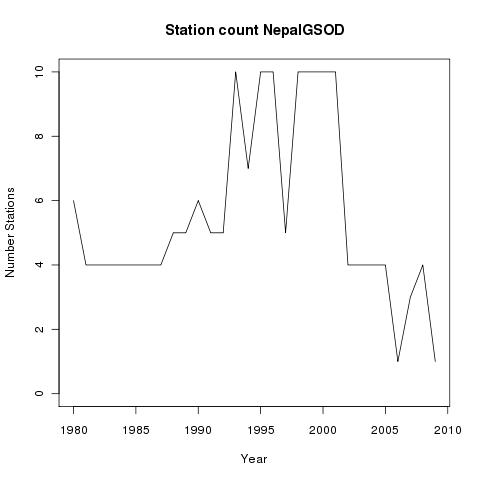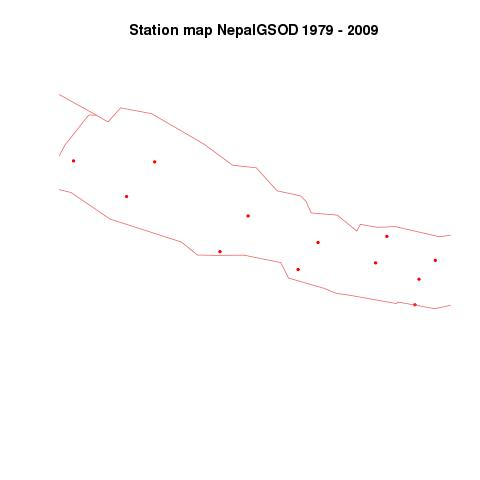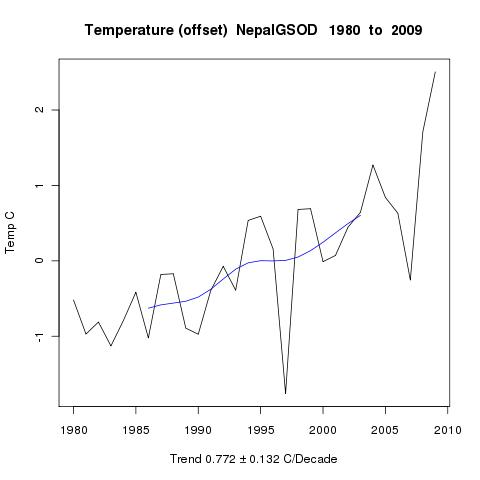#### Himalaya

The next analysis is of the Himalayas generally, defined as anywhere in Nepal, India or Pakistan N of a line given by 14*Lat+8*lon>1046 and above 1000m. For those interested in working TempLS, the specifying expression is:
mask=expression({a=tv\$country;(a==217 | a==219 | a==207) & tv\$alt>1000 & 14*tv\$lat+8*tv\$lon>1046});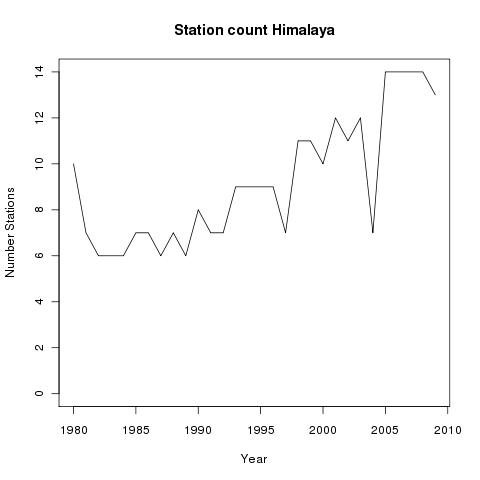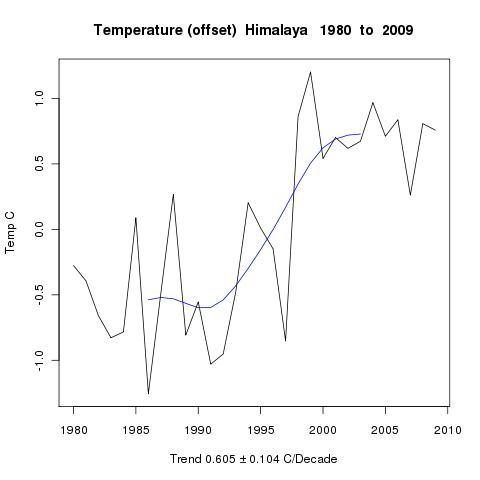#### Trends

Finally, here is the collected table of trends (C/decade) since 1980 and since 1990:

 Trend Nepal Himalaya Number 12 24 1980-2009 0.7747 0.6053 Trend_se 0.132 0.1041 1990-2009 1.003 0.8847 Trend_se 0.2804 0.1832

Here is the earlier set of trends, which used the incorrect input data:
 Trend NepalGSOD HiNepal Himalaya HiHimal Number 12 2 24 7 1980-2009 0.7723 0.7146 0.5036 0.5642 Trend_se 0.1323 0.1438 0.1019 0.1076 1990-2009 1.006 1.018 0.7467 0.8267 Trend_se 0.281 0.3006 0.1947 0.2113

#### Conclusion

Being able to analyse various selected subsets means that we get a feel for how regional variations might affect the conclusion that the area is warming. All these subsets show it to a marked degree. No sub-region has a rate less than 0.5 C/decade (5 deg/century).

Update. Here is a list of the stations in the Himalaya set with some metadata.
urban: A=rural, C=urban, B=small town
country: 207=India 217=Nepal 219=Pakistan
length: Number of years with atleast one month record

 Name country alt startyr endyr length yrs urban lat lon SRINAGAR 207 1587 1973 2010 38 C 34.08 74.83 SHIMLA 207 2202 1973 1973 1 C 31.1 77.17 KULLU MANALI 207 1089 2005 2005 1 A 31.88 77.18 DADELDHURA 217 1865 1978 2001 8 A 29.3 80.58 JUMLA 217 2300 1990 2001 10 A 29.28 82.17 KATHMANDU AIRPORT 217 1337 1976 2010 35 C 27.7 85.37 SYANGBOCHE 217 3700 1976 1979 4 A 27.82 86.72 OKHALDHUNGA 217 1720 1976 2001 12 A 27.3 86.5 TAPLEJUNG 217 1732 1978 2001 11 A 27.35 87.67 DHANKUTA 217 1210 1976 2008 32 A 26.98 87.35 GUPIS 219 2156 2006 2009 4 A 36.17 73.4 CHITRAL 219 1500 1973 2010 22 A 35.85 71.83 DIR 219 1370 1980 2010 12 A 35.2 71.85 DROSH 219 1465 1957 2010 42 A 35.57 71.78 GILGIT 219 1459 1973 2009 11 B 35.92 74.33 SKARDU 219 2181 1975 2009 13 A 35.3 75.68 BUNJI 219 1470 2005 2009 5 A 35.67 74.63 CHILLAS 219 1251 2005 2009 5 A 35.42 74.1 ASTORE 219 2168 2005 2009 5 A 35.37 74.9 CHITRAL 219 1500 2005 2005 1 A 35.88 71.8 BATTAL 219 1676 1975 1976 2 A 34.58 73.15 KAKUL 219 1309 1973 2010 19 C 34.18 73.25 CHERAT 219 1372 2005 2009 5 A 33.82 71.88 MURREE 219 2127 1973 2010 15 C 33.92 73.38